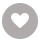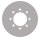# Java 9 Data Structures and Algorithms电子书

45人正在读 | 0人评论9.8

• 读书简介
• 目录
• 累计评论(0条)
• 读书简介
• 目录
• 累计评论(0条)
Gain a deep understanding of the complexity of data structures and algorithms and discover the right way to write more efficient code About This Book ?This book provides complete coverage of reactive and functional data structures ?Based on the latest version of Java 9, this book illustrates the impact of new features on data structures ?Gain exposure to important concepts such as Big-O Notation and Dynamic Programming Who This Book Is For This book is for Java developers who want to learn about data structures and algorithms. Basic knowledge of Java is assumed. What You Will Learn ?Understand the fundamentals of algorithms, data structures, and measurement of complexity ?Find out what general purpose data structures are, including arrays, linked lists, double ended linked lists, and circular lists ?Get a grasp on the basics of abstract data types―stack, queue, and double ended queue ?See how to use recursive functions and immutability while understanding and in terms of recursion ?Handle reactive programming and its related data structures ?Use binary search, sorting, and efficient sorting―quicksort and merge sort ?Work with the important concept of trees and list all nodes of the tree, traversal of tree, search trees, and balanced search trees ?Apply advanced general purpose data structures, priority queue-based sorting, and random access immutable linked lists ?Gain a better understanding of the concept of graphs, directed and undirected graphs, undirected trees, and much more In Detail Java

Java 9 Data Structures and Algorithms

Java 9 Data Structures and Algorithms

Credits

www.PacktPub.com

eBooks, discount offers, and more

Why subscribe?

Customer Feedback

Preface

What this book covers

What you need for this book

Who this book is for

Conventions

Customer support

Errata

Piracy

Questions

1. Why Bother? – Basic

The performance of an algorithm

Best case, worst case and the average case complexity

Analysis of asymptotic complexity

Asymptotic upper bound of a function

Asymptotic upper bound of an algorithm

Asymptotic lower bound of a function

Asymptotic tight bound of a function

Optimization of our algorithm

Fixing the problem with large powers

Improving time complexity

Summary

2. Cogs and Pulleys – Building Blocks

Arrays

Insertion of elements in an array

Insertion of a new element and the process of appending it

Appending at the end

Insertion at the beginning

Insertion at an arbitrary position

Looking up an arbitrary element

Removing an arbitrary element

Iteration

Insertion at the beginning or at the end

Insertion at an arbitrary location

Removing the first element

Removing an arbitrary element

Removal of the last element

Insertion

Removal

Rotation

Summary

3. Protocols – Abstract Data Types

Stack

Fixed-sized stack using an array

Variable-sized stack using a linked list

Queue

Fixed-sized queue using an array

Variable-sized queue using a linked list

Double ended queue

Fixed-length double ended queue using an array

Variable-sized double ended queue using a linked list

Summary

4. Detour – Functional Programming

Recursive algorithms

Lambda expressions in Java

Functional interface

Implementing a functional interface with lambda

The forEach method for a linked list

Fold operation on a list

Filter operation for a linked list

The flatMap method on a linked list

Analysis of the complexity of a recursive algorithm

Performance of functional programming

Summary

5. Efficient Searching – Binary Search and Sorting

Search algorithms

Binary search

Complexity of the binary search algorithm

Sorting

Selection sort

Complexity of the selection sort algorithm

Insertion sort

Complexity of insertion sort

Bubble sort

Inversions

Complexity of the bubble sort algorithm

A problem with recursive calls

Tail recursive functions

Non-tail single recursive functions

Summary

6. Efficient Sorting – quicksort and mergesort

quicksort

Complexity of quicksort

Random pivot selection in quicksort

mergesort

The complexity of mergesort

Avoiding the copying of tempArray

Complexity of any comparison-based sorting

The stability of a sorting algorithm

Summary

7. Concepts of Tree

A tree data structure

The traversal of a tree

The depth-first traversal

The tree abstract data type

Binary tree

Types of depth-first traversals

Non-recursive depth-first search

Summary

8. More About Search – Search Trees and Hash Tables

Binary search tree

Insertion in a binary search tree

Invariant of a binary search tree

Deletion of an element from a binary search tree

Complexity of the binary search tree operations

Self-balancing binary search tree

AVL tree

Complexity of search, insert, and delete in an AVL tree

Red-black tree

Insertion

Deletion

The worst case of a red-black tree

Hash tables

Insertion

The complexity of insertion

Search

Complexity of the search

Summary

9. Advanced General Purpose Data Structures

Heap

Insertion

Removal of minimum elements

Analysis of complexity

Serialized representation

Array-backed heap

Insertion

Removal of the minimal elements

Complexity of operations in ArrayHeap and LinkedHeap

Binomial forest

Why call it a binomial tree?

Number of nodes

The heap property

Binomial forest

Complexity of operations in a binomial forest

Sorting using a priority queue

In-place heap sort

Summary

10. Concepts of Graph

What is a graph?

Representation of a graph in memory

Complexity of operations in a sparse adjacency matrix graph

Complexity of operations in a dense adjacency-matrix-based graph

Complexity of operations in an adjacency-list-based graph

Adjacency-list-based graph with dense storage for vertices

Complexity of the operations of an adjacency-list-based graph with dense storage for vertices

Traversal of a graph

Complexity of traversals

Cycle detection

Complexity of the cycle detection algorithm

Spanning tree and minimum spanning tree

For any tree with vertices V and edges E, |V| = |E| + 1

Any connected undirected graph has a spanning tree

Any undirected connected graph with the property |V| = |E| + 1 is a tree

Cut property

Minimum spanning tree is unique for a graph that has all the edges whose costs are different from one another

Finding the minimum spanning tree

Union find

Complexity of operations in UnionFind

Implementation of the minimum spanning tree algorithm

Complexity of the minimum spanning tree algorithm

Summary

11. Reactive Programming

What is reactive programming?

Producer-consumer model

Semaphore

Compare and set

Volatile field

Producer-consumer implementation

Spinlock and busy wait

Functional way of reactive programming

Summary

Index

01

￥122.99
02

Vijay Kumar Velu
￥153.00
03

Victor Wu
￥153.00
04

Bill Kastanakis
￥122.00
05

Andrew J Wagner
￥122.00
06

Tony Campbell
￥92.00
07

￥138.00
08

Lee Allen
￥184.00
09

Greg Lukosek
￥138.00
10

### R Machine Learning By Example￥153.00

Raghav Bali
￥153.00
•关注我们

最受欢迎的阅读产品

关注我们：

•关于我们

欢迎反馈宝贵意见给我们

客服书吧：当当云阅读问答